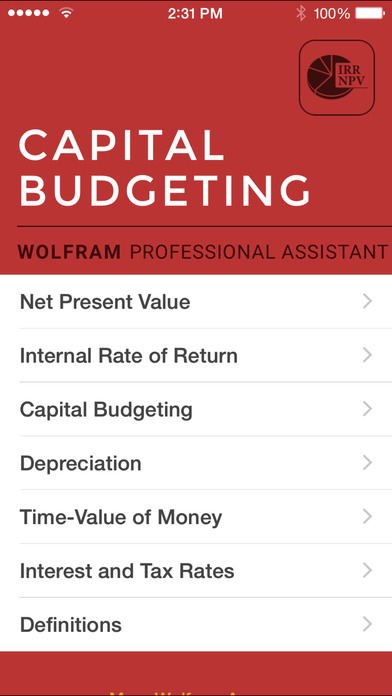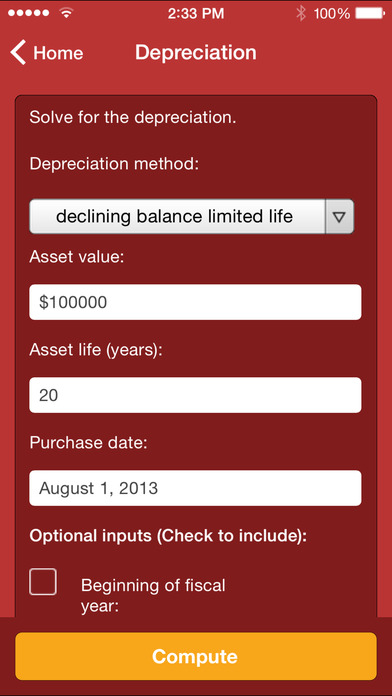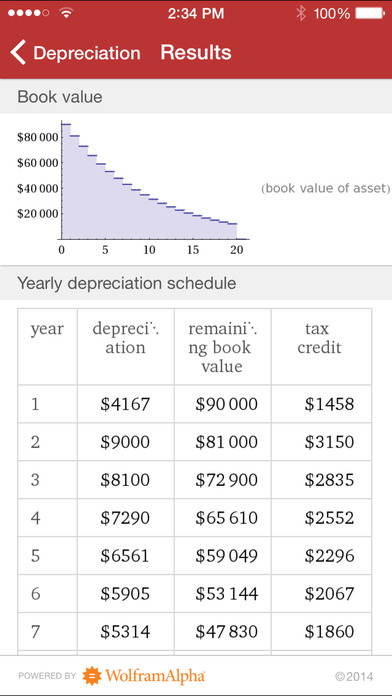# Wolfram Capital Budgeting Professional Assistant

## iOS UniversalFinance

\$2.99

When you need information about planning your long-term business investments, the Wolfram Capital Budgeting Professional Assistant will guide your decisions with calculators for depreciation, economic value added, rates of return, annuity values, and much more. This app provides accurate tools for computing what you need when it comes to capital budgeting, such as net present value (NPV), internal rate of return (IRR), and discounted cash flow (DCF).

- Solve for the capital recovery factor and interest rate using interest periods or compounding periods
- Use the economic value added to calculate the net operating profit after taxes and the weighted average cost of capital
- Get results for computations like the equivalent annual cost, profitability index, real rate of return, and return on investment
- Get detailed results for weighted costs of capital like cost of equity and debt, tax rate, total equity, and debt
- Solve for depreciation with various depreciation methods like straight line, declining balance, accelerated, sum of years digits, and more
- Compute present and future value, interest rate, compounding periods, periodic payments, and periodic payment growth rate by using ordinary annuities or annuities due
- Research current interest rates on credit cards, mortgage refinance, personal loans, long-term interest rates, LIBOR rates, and others
- Do calculations for lump sums, nominal and effective rates, and amortization

The Wolfram Capital Budgeting Professional Assistant is powered by the Wolfram|Alpha computational knowledge engine and is created by Wolfram Research, makers of Mathematica—the world's leading software system for mathematical research and education.

The Wolfram Capital Budgeting Professional Assistant draws on the computational power of Wolfram|Alpha's supercomputers over a 3G, 4G, or Wi-Fi connection.

## What's New

- Bug fixes

•••••